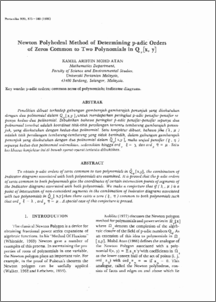# Newton Polyhedral Method of Determining p-adic Orders of Zeros Common to Two Polynomials in Qp[x, y]

## Citation

Mohd Atan, Kamel Ariffin (1986) Newton Polyhedral Method of Determining p-adic Orders of Zeros Common to Two Polynomials in Qp[x, y]. Pertanika, 9 (3). pp. 375-380.

## Abstract

To obtain p-adic orders of zeros common to two polynomials in Q [x,y], the combination of P . Indicator diagrams assodated with both polynomials are examined. It is proved that the p-adic orders of zeros common to both polynomials give the coordinates of certain intersection points of segments of the Indicator diagrams assodated with both polynomials. We make a conjecture that if ( A, IJ. ) is a point of intersection of non-coinddent segments in the combination of Indicator diagrams associated with two polynomials in Q [ x,y l then there exists a zero (L Tl) common to both polynomials such that ord ~. = A , ord Tl::: IJ. . A special case of this conjecture is proved.Preview
PDFView Item